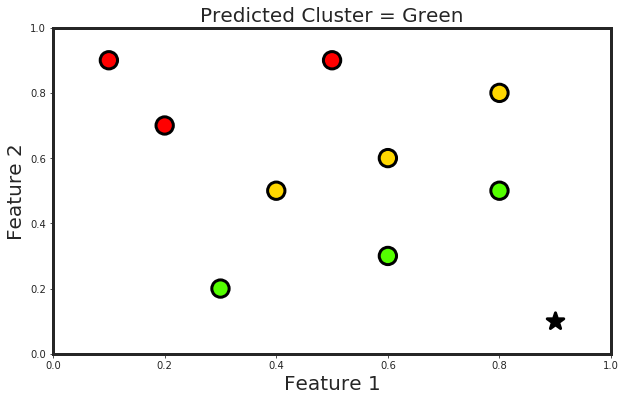## In a PNN, the operations are organized into a multilayered feedforward network with four layers:

• Input layer
• Pattern layer
• Summation layer
• Output layer
``````# Loading Libraries
import numpy as np
import pandas as pd
import matplotlib.pyplot as plt
import matplotlib as mpl
import seaborn as sns
sns.set_style("ticks")
mpl.rcParams['axes.linewidth'] = 3
mpl.rcParams['lines.linewidth'] =7
``````
``````def Create_DataFrame():
# defining the features and class labels as a dictionary
data = {
"Feature_1" : [0.1, 0.5,  0.2, 0.6,  0.8,  0.4,  0.8,  0.6,  0.3],
"Feature_2" : [0.9, 0.9,  0.7, 0.6,  0.8,  0.5,  0.5,  0.3,  0.2],
"Class_Label" : [1, 1, 1, 2, 2, 2, 3, 3, 3]
}

# converting the dictionary into a dataframe
df = pd.DataFrame(data = data)

return df
``````
``````def PNN(df, DesiredPoint):
# defining a group for each class labels
Clusters = df.groupby("Class_Label")

# defining the number of classes as clusters
NumClusters = len(Clusters)

# an empty dictionary for calculating the sum of Gaussian for each class
GaussianSums = dict()

# defining the number of features
NumFeatures = df.shape - 1

# defining the standard deviation for Gaussian distribution
Sigma = 1.0

# creating features array
Features = df.drop(["Class_Label"], axis = 1).values

# INPUT LAYER OF PNN

# defining a row variable for moving over the data row by row
_row = 0

# loop over the number of clusters
for i in range(1, NumClusters + 1):

# initialize the GaussianSum for each class
GaussianSums[i] = 0.0

# defining the number of points per cluster
PointsPerCluster = len(Clusters.get_group(i))

# PATTERN LAYER OF PNN
# definining temporary sum for holding the sum of X and Y elements
TempSum = 0.0
# loop over points of each cluster and GaussianSum calculation
for j in range(1, PointsPerCluster + 1):
# calculating the X element of Gauassian
TempX = ( DesiredPoint - Features[_row]  )**2
# calculating the y element of Gauassian
TempY = ( DesiredPoint - Features[_row]  )**2
# calculating the Gaussian
TempCoeff = -(TempX + TempY)/(2.0 * Sigma**2)
# adding the calculated Gaussian for all the points per cluster
TempSum +=  TempCoeff
# incrementing the row to cover all points per cluster
_row += 1
# storing the GaussianSum per cluster in a dictionary
GaussianSums[i] = TempSum

# returning the key of the maximum GaussianSum per cluster
CalculatedClass = max(GaussianSums, key = GaussianSums.get)

# Visualization
Visualization(df, Features, DesiredPoint, CalculatedClass)

print("Calculated Class = " + str(CalculatedClass))
``````
``````def Visualization(df, Features, DesiredPoint, CalculatedClass):

color_dict = {1 : "Red", 2 : "Yellow", 3 : "Green"}
plt.figure(figsize=(10,6))
plt.scatter(Features[:,0],
Features[:,1],
s = 300.,
c = df["Class_Label"],
cmap=plt.cm.prism,
marker = "o",
lw = 3,
edgecolor='k')
plt.scatter(DesiredPoint,
DesiredPoint,
s = 300.,
c = "k",
marker = "*",
lw = 3,
edgecolor='k')
plt.xlabel("Feature 1", fontsize = 20)
plt.ylabel("Feature 2", fontsize = 20)
plt.title("Predicted Cluster = " + color_dict[CalculatedClass], fontsize = 20)
plt.xlim([0,1])
plt.ylim([0,1])
plt.show()
``````
``````def main():
# desired point for clustering
DesiredPoint = [0.9, 0.1]
df = Create_DataFrame()
print("************** Dataframe ***************")
print(df)
print("****************************************")
print("Desired Point = [{}, {}]".format(DesiredPoint, DesiredPoint))
PNN(df, DesiredPoint)
``````
``````main()
``````
``````************** Dataframe ***************
Class_Label  Feature_1  Feature_2
0            1        0.1        0.9
1            1        0.5        0.9
2            1        0.2        0.7
3            2        0.6        0.6
4            2        0.8        0.8
5            2        0.4        0.5
6            3        0.8        0.5
7            3        0.6        0.3
8            3        0.3        0.2
****************************************
Desired Point = [0.9, 0.1]
````````````Calculated Class = 3
``````